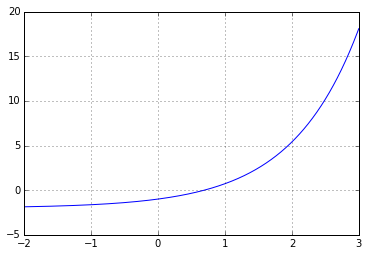# Convergence of Newton's Method¶

In :
import numpy as np
import matplotlib.pyplot as pt

In :
def f(x):
return np.exp(x) - 2

In :
xgrid = np.linspace(-2, 3, 1000)
pt.grid()
pt.plot(xgrid, f(xgrid))

Out:
[<matplotlib.lines.Line2D at 0x7f44dd2925c0>]What's the true solution of $f(x)=0$?

In :
xtrue = np.log(2)
print(xtrue)
print(f(xtrue))

0.69314718056
0.0


Now let's run Newton's method and keep track of the errors:

In :
errors = []
x = 2
xbefore = 3


At each iteration, print the current guess and the error.

In :
slope = (f(x)-f(xbefore))/(x-xbefore)

xbefore = x
x = x - f(x)/slope
print(x)
errors.append(abs(x-xtrue))
print(errors[-1])

nan
nan

-c:1: RuntimeWarning: invalid value encountered in double_scalars

In :
for err in errors:
print(err)

0.882400077493
0.411823511031
0.147482044876
0.0276859623403
0.00198268429064
2.73106724006e-05
2.70651508982e-08
3.69593244898e-13
1.11022302463e-16
1.11022302463e-16
nan

• Do you have a hypothesis about the order of convergence?
In :
# Does not quite double the number of digits each round--unclear.


Let's check:

In :
for i in range(len(errors)-1):
print(errors[i+1]/errors[i]**1.618)

0.504224909965
0.619635842142
0.612688428557
0.657169643929
0.644727394358
0.655276572424
0.648759771781
14482.1405299
7243300082.99
nan